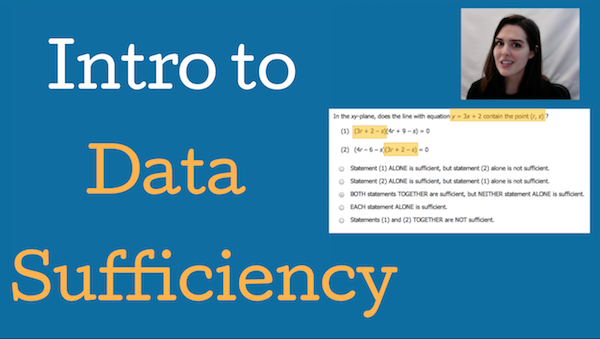# Online Lesson: Introduction to GMAT Data Sufficiency [Video]Many students think that Data Sufficiency is one of the hardest question types on the GMAT. Data Sufficiency is unlike anything they’ve ever seen before, either on tests or in their undergraduate math classes. In “Online Lesson: Introduction to GMAT Data Sufficiency”, we cover the basics of GMAT Data Sufficiency to familiarize you with your new favorite GMAT question type and give you strategies you can apply on test day.

Since this Introduction to GMAT video is relatively long, we’ve broken it down into bite-sized sections focused on key Data Sufficiency topics:

In Overview of GMAT Quant, we discuss the makeup of the GMAT Quantitative section, answering the question “How many Data Sufficiency questions are there on the GMAT?” In Structure of Data Sufficiency questions, we review the three components of a Data Sufficiency problem (the question stem, the statements, and the answer choices) and discuss Data Sufficiency GMAT tips related to this structure. We then delve into the answer choices with What is Sufficiency?”by discussing what the “sufficiency” in GMAT Data Sufficiency means and looking at the two different kinds of sufficiency we see in Data Sufficiency questions.

Once we’ve covered the foundations of GMAT Data Sufficiency, we take a detour for What’s the Point of Data Sufficiency? to discuss how Data Sufficiency problems test skills critical to success in business school and careers. We then discuss How to Approach Data Sufficiency Questions at a broad level, walking chronologically through the steps test takers can apply on every Data Sufficiency problem and providing additional GMAT Data Sufficiency tips. Finally, we walk through more specific Data Sufficiency GMAT Strategy in Data Sufficiency Strategies, covering four of the most useful strategies for GMAT Data Sufficiency.